# Minkowski inequality

Jump to: navigation, search

The proper Minkowski inequality: For real numbers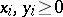,, and for,(1)

This was derived by H. Minkowski . For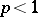,, the inequality is reversed (forone must have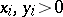). In each case equality holds if and only if the rows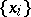and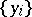are proportional. For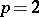Minkowski's inequality is called the triangle inequality. Minkowski's inequality can be generalized in various ways (also called Minkowski inequalities). Below some of them are listed.

Minkowski's inequality for sums. Let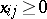forand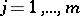and let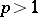. Then(2)

The inequality is reversed for,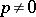, and for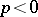it is assumed that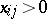. In each case equality holds if and only if the rows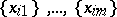are proportional. There are also generalizations of

for multiple and infinite sums. However, when limit processes are used special attention is required in formulating the case of possible equality (see ).

Inequalities

and (2) are homogeneous with respect to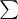and therefore have analogues for various means, for example, if, where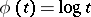, then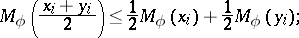for more details see .

Minkowski's inequality for integrals is similar to

and also holds because of the homogeneity with respect to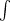. Letbe integrable functions in a domain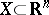with respect to the volume element. Then for,(3)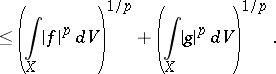A generalization of (3) to more general functions can be obtained naturally. A further generalization is: If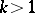, then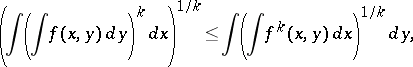where equality holds only if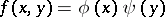.

Other inequalities of Minkowski type:

a) for products: If, thenb) Mahler's inequality: Let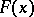be a generalized norm on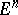andits polar function; thenwhere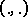is the inner product;

c) for determinants: If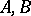are non-negative Hermitian matrices over, thenFinally, connected with the name of Minkowski are other inequalities; in particular, in convex analysis and number theory. For example, the Brunn–Minkowski theorem.